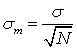### Why should I choose AnalystNotes?

Simply put: AnalystNotes offers the best value and the best product available to help you pass your exams.

##### Subject 3. Standard Error of the Sample Mean
The standard error of a statistic is the standard deviation of the sampling distribution of that statistic.

Standard errors are important because they reflect how much sampling fluctuation a statistic will show. The inferential statistics involved in the construction of confidence intervals and significance testing are based on standard errors. The standard error of a statistic depends on the sample size. In general, the larger the sample size, the smaller the standard error. The standard error of a statistic is usually designated by the Greek letter sigma (σ) with a subscript indicating the statistic.

The standard error of the mean is designated as: σm. It is the standard deviation of the sampling distribution of the mean. The formula for the standard error of the mean is:where σ is the standard deviation of the original distribution and N is the sample size (the number of scores each mean is based upon).

This formula does not assume a normal distribution. However, many of the uses of the formula do assume a normal distribution. The formula shows that the larger the sample size, the smaller the standard error of the mean. More specifically, the size of the standard error of the mean is inversely proportional to the square root of the sample size.

Example 1

Suppose that the mean grade of students in a class is 62%, with a standard deviation of 10%. A sample of 30 students is taken from the class. Calculate the standard error of the sample mean and interpret your results.

You are given that μ = 62, and σ =10. Since n = 30, the standard error of the sample mean is: σm = 10/301/2 = 1.8257. This means that if you took all possible samples of size 30 from the class, the mean of all those samples would be 62 and the standard error would be 1.8257.

Note that if you took a sample size of 50, the standard error would then be: σm = 10 / 501/2 = 1.4142.

The standard error would drop as the sample size increased, which agrees with the information above.

When sample standard deviation (s) is used as an estimate of σ (when it is unknown), the estimated standard error of the mean is s/N1/2. In most practical applications analysts need to use this formula because the population standard deviation is almost never available.

Example 2

Suppose that the mean grade of students in a class is unknown, but a sample of 30 students is taken from the class and the mean from the sample is found to be 60%, with a standard deviation of 9%. Calculate the standard error of the sample mean and interpret your results.

Now, μ and σ are unknown, but m is given as 60 and s is given as 9. Since n = 30, you can estimate the standard error of the sample mean as: 9/301/2 = 1.6432. This means that if you took all possible samples of 30 from the class, you would estimate the standard error to be 1.6432.

It is important to note that when you have σ, you must use it; when you don't, you use its sample equivalent, s.

User Comment
achu std error = f(s, n)
jpducros Standart error formula is independant from the shape of the distribution (standart or not)....important...
polanki If S is given to sample which is standard deviation of sample or standard error then why again we need to devide it by sqrt N to get sample error.
munro polanki: it's the lowercase s, nor the uppercase S.
Raok may I ask how to differentiate between sample size and number of sample, isn't in this case, N is sample not same size?
jayj001 SAMPLE SIZE = no. of observations in each sample

e.g. 100 samples with 5 observations in each

NOT normal distribution as N < 30
fanDango You can have a sample size of 30 students from a population of 1000 students and also have 100 samples of 30 students. 100 is the number of samples, 30 is the sample size.
Bududeen it depends if the distribution itself is not normal...if the distribution is normal , then the sample size does not affect the normality of the distribution
so even with a sample size of 5, if the distribution is normal then the distribution follows a normal dist.
GouldenOne What is variable X?
sgossett86 Basically we're navigating from initial statistic theory to how to take our own imperfect data and make standard probability distributions so we can standardize and make inferences comparatively as an analyst.
Rachelle3 its always standard deviation divided by sample (of n) I get that but when you know this then you always do it the power of 0.5?
mcbreatz Power 0f .5 is same as taking the square root which is specified in the formula.